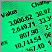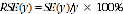6227.0.30.001 - Microdata: Education and Work, Australia, May 2011
ARCHIVED ISSUE Released at 11:30 AM (CANBERRA TIME) 15/05/2012
 Page tools: .mffeedback,#pjs { display :none; } Enable Javascript to Print PagesPrint AllFILE STRUCTURE WEIGHTS AND ESTIMATION STANDARD ERRORS NOT APPLICABLE CATEGORIES POPULATIONS WEIGHTS AND ESTIMATION As the survey was conducted on a sample of households in Australia, it is important to take account of the method of sample selection when deriving estimates. This is particularly important as a person's chance of selection in the survey varied depending on the state or territory in which they lived. Survey 'weights' are values which indicate how many population units are represented by the sample unit. There is one weight provided: a person weight. This should be used when analysing data at the person level. Where estimates are derived, it is essential that they are calculated by adding the weights of persons in each category, and not just by counting the number of records falling into each category. If each person's 'weight' were to be ignored, then no account would be taken of a person's chance of selection in the survey or of different response rates across population groups, with the result that counts produced could be seriously biased. The application of weights ensures that the person estimates conform to an independently estimated distribution of the population by age, sex, state/territory, part of state and labour force status.Back to top STANDARD ERRORS Each record on the person level also contains 30 replicate weights and, by using these weights, it is possible to calculate standard errors for weighted estimates produced from the microdata. This method is known as the 30 group Jack-knife variance estimator. Under the Jackknife method of replicate weighting, weights were derived as follows: 30 replicate groups were formed with each group formed to mirror the overall sample (where units from a collection district all belong to the same replicate group and a unit can belong to only one replicate group) one replicate group was dropped from the file and then the remaining records were weighted in the same manner as for the full sample records in that group that were dropped received a weight of zero. This process was repeated for each replicate group (i.e. a total of 30 times). Ultimately each record had 30 replicate weights attached to it with one of these being the zero weight. Replicate weights enable variances of estimates to be calculated relatively simply. They also enable unit records analyses such as chi-square and logistic regression to be conducted which take into account the sample design. Replicate weights for any variable of interest can be calculated from the 60 replicate groups, giving 60 replicate estimates. The distribution of this set of replicate estimates, in conjunction with the full sample estimate (based on the general weight) is then used to approximate the variance of the full sample. To obtain the standard error of a weighted estimate y, the same estimate is calculated using each of the 60 replicate weights. The variability between these replicate estimates (denoting y(g) for group number g) is used to measure the standard error of the original weighted estimate y using the formula:where: g = the replicate group number y(g) = the weighted estimate, having applied the weights for replicate group g y = the weighted estimate from the sample. The 30 group Jack-knife method can be applied not just to estimates of the population total, but also where the estimate y is a function of estimates of the population total, such as a proportion, difference or ratio. For more information on the 30 group Jack-knife method of SE estimation, see Research Paper: Weighting and Standard Error Estimation for ABS Household Surveys (Methodology Advisory Committee), July 1999 (cat. no. 1352.0.55.029). Use of the 30 group Jack-knife method for complex estimates, such as regression parameters from a statistical model, is not straightforward and may not be appropriate. The method as described does not apply to investigations where survey weights are not used, such as in unweighted statistical modelling.Back to top NOT APPLICABLE CATEGORIES Most data items included in the microdata include a 'Not applicable' category. The classification value of the 'Not applicable' category, where relevant, are shown in the data item lists in the Downloads tab.Back to top POPULATIONS The population relevant to each data item is identified in the data item list and should be borne in mind when extracting and analysing data from the CURF or in TableBuilder. The actual population count for each data item is equal to the total cumulative frequency minus the 'Not applicable' category. Generally all populations, including very specific populations, can be 'filtered' using other relevant data items. For example, if the population of interest is 'Employed persons', any data item with that population (excluding the 'Not applicable' category) can be used. For example, the CURF data items 'Status in employment in current job' (SWORKER) or 'Industry (ANZSIC 2006)' (INDCUR) are applicable to employed persons only. Therefore, either of the following filters could be used when restricting a table to 'Employed persons' only: SWORKER > 0 or INDCUR < 26 (Note: For those data items, the 'Not applicable' categories (i.e. those persons who are not employed) are codes 0 and 26 respectively and would be excluded from either population filter shown above.) Conversely, code 1 for the data items 'Labour force status' (LFSTATC) is 'employed persons'. Therefore, once again if the population of interest is employed persons, this data item could be used as the filter (i.e. LFSTATC = 1). Document Selection These documents will be presented in a new window.